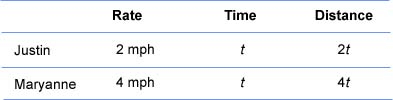Distance and Motion Problems

Solving Motion Problems Involving Two Rates

A motion problem is one in which an object is moving at a specific rate for a specific period of time. Motion problems are often solved by using the distance formula:

distance = rate x time

In motion problems that involve two rates, such as two trains traveling different speeds, let the variable represent one of the unknown quantities and then represent the second quantity in terms of the first.

Example

Justin and Maryanne both rent kayaks to paddle down the river. Both kayaks start at the same time from the same point and travel in the same direction. Justin paddles his kayak at 2 miles per hour, and Maryanne paddles her kayak at 4 miles per hour. In how many hours will the two kayaks be 5 miles apart?

Understand
The question asks to find the time it takes for the kayaks to be separated by 5 miles.

Let t = the time when kayaks are 5 miles apart.

The chart below establishes the rate, time, and distance for each kayak.Since the kayaks are traveling in the same direction, the distance between them is found by subtracting the distance traveled by the slower kayak from the distance traveled by the faster kayak.

Translate

(distance traveled by faster kayak) - (distance traveled by slower kayak) = 5 miles

4t - 2t = 5 miles
Solve
4t - 2t = 5
2t = 5
t = 2.5